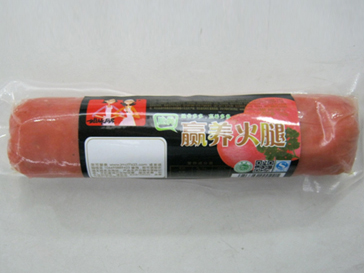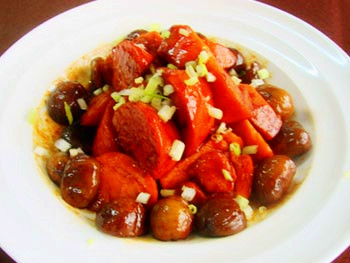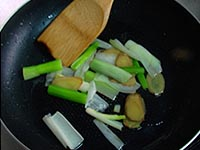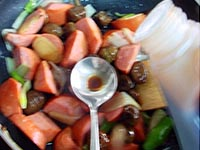﻿ 脆骨赢养火腿 - 消费食品 - 姐妹厨房 享受安心的味道 ﻿
• 鸡柳类
• 香嫩鸡柳
• 川香鸡柳
• 金三角鸡柳
• 汉堡类
• 汉堡
• 老虎饼
• 牛肉汉堡
• 牛肉饼
• 乡美牛肉饼
• 乡美汉堡
• 五香鸡肉饼
• 超厚鸡排
• 猪肉贝帝堡（原味）
• 脆香猪排
• 甜块类
• 芒果慕斯
• 奶油玉米甜心
• 丸子类
• 御膳丸
• 四喜御膳丸
• 月亮烧
• 包心丸子
• 红烧丸子
• 原肉类
• 鸡腿汉堡
• 香鸡排
• 龙田扬
• 翅类
• 吮指炸翅根
• 吮指炸翅中
• 夹心类
• 黄金翡翠鸡派
• 台畜类
• 三明治切片
• 德式香肠
• 培根
• 香薰鸡肉片
• 台湾脆骨肠
• 土鸡类
• 香醉土鸡翅
• 香葱土鸡腿
• 香醇白斩土鸡
• 原汁土鸡汤
•• 冷冻鸡肉
• 急鲜封鸡胸肉
• 急鲜封琵琶腿
• 急鲜封翅中
• 急鲜封翅根
• 急鲜封滑嫩鸡胸肉
• 急鲜封滑嫩琵琶腿
• 飞雪土鸡
• 飞雪土鸡散养鸡
• 飞雪土鸡鸡汤
• 飞雪土鸡翅根
• 飞雪土鸡翅中尖
• 飞雪土鸡琵琶腿
• 飞雪土鸡鸡柳
• 飞雪土鸡大胸
• 鸡肉肠
• 台式鸡肉肠
• 鸡肉烤肠
• 鸡肉蒜肠
• 鸡肉红肠
• 德式鸡肉肠
• 维也纳鸡肉肠
• 台湾风味脆骨肠
• 鸡肉火腿
• 法式鸡肉火腿
• 意式鸡肉火腿
• 脆骨赢养火腿
• 鸡肝赢养火腿
• 台畜猪肉系列
• 牧风原肉火腿
• 熏腿原肉火腿
• 古早味切片火腿
• 三明治切片火腿
• Q弹火腿
• 德式香肠
• 台湾米肠
• 扬州炒饭
• 台式油饭
• 海南鸡饭
• 海南鸡饭情侣套装
• 扬州炒饭情侣套装
• 台式油饭情侣套装
• 海盗肠
• 奥尔良
• 原味
• 照烧
• 泰式甜辣
• 脆骨肠
• 台式
• 蒜香
• 奥尔良
• 香辣
• 糖熏卤味
• 糖熏鸡腿
• 糖熏鸡脖
• 糖熏鸡爪
• 阿拉善沙漠节水小米
• 谷物礼盒
•• 品 名

脆骨赢养火腿• 净 含 量

250g• 口 味

原味• 烹调方法

开袋即食可加热• 订购热线

400-810-9066•精选有身份证的安心鲜鸡肉，加入鸡脆骨，经五星级酒店西餐主厨精心调制出口感香脆，真是脆骨多多，赢养多多。烧栗子赢养火腿赢养火腿（切滚刀块）、烧汁、酱油、生姜、京葱、小葱花、栗子、鸡汤炒锅内下少许油，爆香姜、葱，下切好的火腿块，稍炒；在1锅内下酱油、烧汁炒至上色，下鸡汤，烧沸，小火收至汁将干时，撒入葱花装盘即可。• 鸡肉
• 鸡胸软骨
• 大豆分离蛋白
• 麦芽糖浆
• 大豆油
• 白砂糖
• 食盐
• 玉米淀粉
• 香辛料
• 食品添加剂